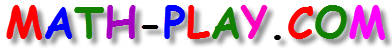#When playing this one digit addition game, kids will add single-digit numbers and match the sums with different addition problems. This cool game is a lot of fun and challanges students to pair all numbers as quickly as possible.

This concentration game provides students with a fun way to practice addition facts. The game can be played on computers, iPads, and other tablets. You do not need to install an app to play this game on the iPad.

The game is based on the following 1st grade math standards:
CC1.OA.3
Apply properties of operations as strategies to add and subtract.
CC1.OA.6
Add and subtract within 20, demonstrating fluency for addition and subtraction within 10. Use strategies such as counting on; making ten (e.g., 8 + 6 = 8 + 2 + 4 = 10 + 4 = 14); decomposing a number leading to a ten (e.g., 13 – 4 = 13 – 3 – 1 = 10 – 1 = 9); using the relationship between addition and subtraction (e.g., knowing that 8 + 4 = 12, one knows 12 – 8 = 4); and creating equivalent but easier or known sums (e.g., adding 6 + 7 by creating the known equivalent 6 + 6 + 1 = 12 + 1 = 13).